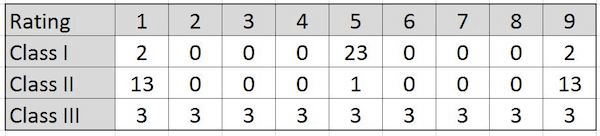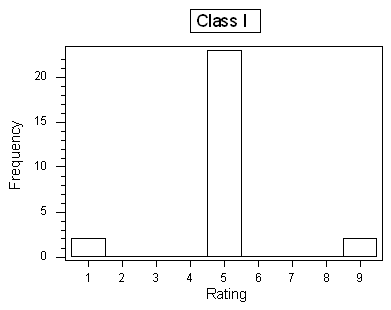## Assignment: Standard Deviation

The concept of standard deviation is less intuitive as a measure of spread than the range or the IQR. The following activity is designed to help you develop a better intuition for the standard deviation.

### Background

At the end of a statistics course, students in three different classes rated their instructor on a number scale of 1 to 9 (1 being “very poor,” and 9 being “best instructor I’ve ever had”). The following table provides three hypothetical rating data:And here are the histograms of the data:### Question 1:

Assume that the average rating in each of the three classes is 5 (which should be visually reasonably clear from the histograms), and recall the interpretation of the SD as a “typical” or “average” distance between the data points and their mean. Judging from the table and the histograms, which class would have the largest standard deviation, and which one would have the smallest standard deviation? Explain your reasoning.

Now check your intuition by finding the actual standard deviations of the three rating distributions.

### Instructions

Click on the link corresponding to your statistical package to see instructions for completing the activity, and then answer the questions below.

### Question 2:

What are the standard deviations of the three rating distributions? Was your intuition correct?